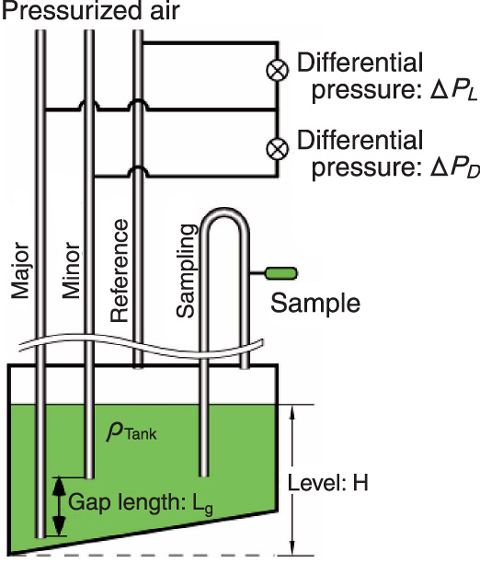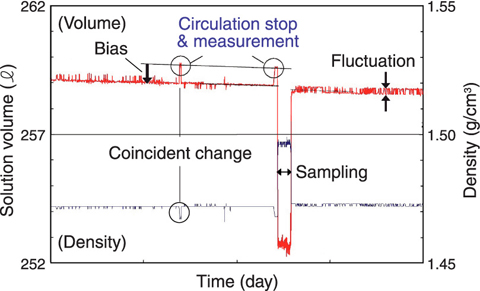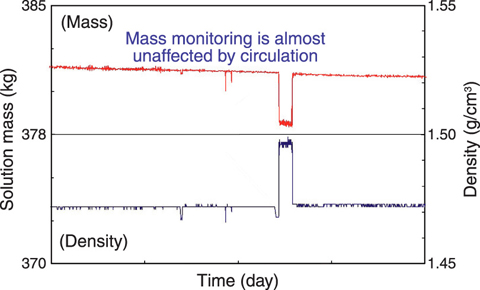# 8-1 Accurate Measurement of Plutonium Nitrate Solution

## - Accuracy & Reliability by Digital-Quartz Transducers Confirm ed by Comparison of Two Dens ities and by Applyin g Th em to Soluti on Mass Monitoring -Fig.8-2 Measurement of solution density and volume in a tankFig.8-3 Example of continuous volume monitoring dataFig.8-4 Example of continuous mass monitoring data

Spent nuclear fuel used in the light water reactor is dissolved and reusable uranium and plutonium are extracted in the form of nitrate solution at the Tokai reprocessing plant. These solutions have to be converted to mixed oxide (MOX) powder to reuse them as nuclear fuel. We have developed and adopted a method to convert directly the mixed nitrate solution to MOX, which has high resistivity to nuclear proliferation because it is absolutely impossible to make pure plutonium oxide. Considering the danger of pure plutonium nitrate solution, we have carried out very carefully and strictly the measurement and management of plutonium.

Plutonium solution before mixing with uranium solution is stored in a tank shown in Fig.8-2. The difference of back pressures between three tubes filled with air is measured accurately using two digital-quartz pressure transducers. The differential pressure between the Major and the Minor tubes (ΔPD), the differential pressure between the Major and the Reference tubes (ΔPL) and the solution volume (V) are expressed in terms of the solution density (ρTank), gravity acceleration (g), solution level (H), vertical differential length between the Major and the Minor (Lg) and the tank-specific (fixed) calibration function (F) to determine V from H:

・ ΔPD = ρTank・g・Lg

・ ΔPL = ρTank・g・H

・ V = F ( H)

Lg and g are constants, so ρTank, H, and V can be calculated from ΔPD and ΔPL. These equations are derived from measurement principles, and actual equations include some corrections for such factors as pressure distribution inside the tubes. It is very important that accurate measurement is done while the solution is motionless. Plutonium mass is det ermined from ρTank and V measured by this method, ρLabo, the solution density and the plutonium concentration of the test sample at the temperature of the laboratory measured by another method. These two density results are corrected for the temperature difference between tank and sample. These results should match after considering the temperature dependency. If the results do not match, it is assumed that there is/are unexpected error/s. In such a case, re-measurement is carried out fully or partly in order to complete accurate determination of plutonium mass.

However, the solution has to be circulated by air to remove hydrogen gas generated by alpha radiation except at the time of accurate precise measurement. During the circulation, a small change in volume caused by motion of solution or change in temperature is observed. Here, we introduced solution mass defined as ρTank × V, and it became clear that we can distinguish clearly the change in volume by appearance and the correct change such as evaporation.

An example of continuous volume monitoring is shown in Fig.8-3. The time when circulation is stopped is very short, and this volume is correct. The air affects ΔPD measurement, and ρTank, causes both the changed appearance of H and the minus bias of around 0.2% and fluctuation of V.

Another example of continuous mass monitoring is shown in Fig.8-4, where the effect of ρTank observed in Fig.8-3 is amazingly reduced. In addition, gradual decrease of mass due to evaporation is observed correctly and it was confirmed that there was little change in volume due to temperature before/ after sampling.

As a result, it is possible to measure correctly and stably the amount of solution using this continuous mass monitoring method even though there is circulation.

Reference
Hosoma, T., Mukai, Y. et al., Reduction of Fluctuation and Small Bias Observed in Continuous Volume Monitoring taken in an Annular Tank for Plutonium Nitrate, Symposium on International Safeguards: Addressing Verification Challenges, (IAEA-CN-148), Vienna, Austria, 2006, p.192-194.

| | | | |